JAJSCS0C December   2016  – January 2018

PRODUCTION DATA.

1. 特長
2. アプリケーション
3. 概要
4. 改訂履歴
5. Device Comparison Table
6. Pin Configuration and Functions
7. Specifications
8. Parameter Measurement Information
9. Detailed Description
1. 9.1 Overview
2. 9.2 Functional Block Diagram
3. 9.3 Feature Description
4. 9.4 Device Functional Modes
10. 10Application and Implementation
1. 10.1 Application Information
2. 10.2 ON and OFF Control
3. 10.3 Input Capacitor (Optional)
4. 10.4 Output Capacitor (Optional)
5. 10.5 Typical Application
11. 11Power Supply Recommendations
12. 12Layout
13. 13デバイスおよびドキュメントのサポート
1. 13.1 デバイス・サポート
2. 13.2 ドキュメントのサポート
3. 13.3 ドキュメントの更新通知を受け取る方法
4. 13.4 コミュニティ・リソース
5. 13.5 商標
6. 13.6 静電気放電に関する注意事項
7. 13.7 Glossary
14. 14メカニカル、パッケージ、および注文情報

• DRV|6
• DBV|6
• DRV|6

#### 10.5.2.1 Shutdown Sequencing During Unexpected Power Loss

Using the adjustable Quick Output Discharge function of the TPS22810, adding a load switch to each power rail can be used to manage the power down sequencing in the event of an unexpected power loss (that is battery removal). To determine the QOD values for each load switch, first confirm the power down order of the device you wish to power sequence. Be sure to check if there are voltage or timing margins that must be maintained during power down. Next, consult Table 1 to determine appropriate CL and RQOD values for each power rail's load switch so that the load switches' fall times correspond to the order in which they need to be powered down. In the above example, we must have this power rail's fall time to be 4 ms. Using Equation 2, we can determine the appropriate RQOD to achieve our desired fall time.

Since fall times are measured from 90% of VOUT to 10% of VOUT, using Equation 2, we get Equation 4 and Equation 5.

Equation 4.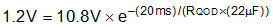Equation 5. RQOD = 413.7 Ω

Consulting Figure 6, RPD at VIN = 12 V is approximately 250 Ω. Using Equation 1, the required external QOD resistance can be calculated as shown in Equation 6 and Equation 7.

Equation 6. 413.7 Ω = 250 Ω + REXT
Equation 7. REXT = 163.7 Ω

Figure 22 through Figure 25 are scope shots demonstrating an example of the QOD functionality when power is removed from the device (both ON and VIN are disconnected simultaneously). In the scope shots, the VIN = 12 V and correspond to when RQOD = 1000 Ω, RQOD= 500 Ω, and QOD = VOUT with two values of CL = 10 µF and 22 µF.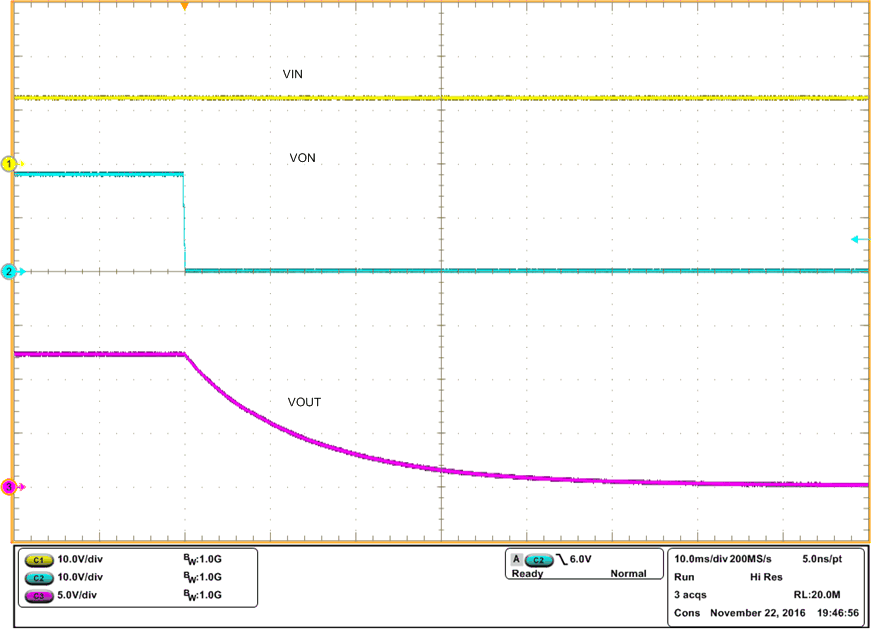VIN = 12 V CIN = 1 µF CL = 10 µF
Figure 22. Fall Time tF at VIN = 12 V, RQOD = 1000 Ω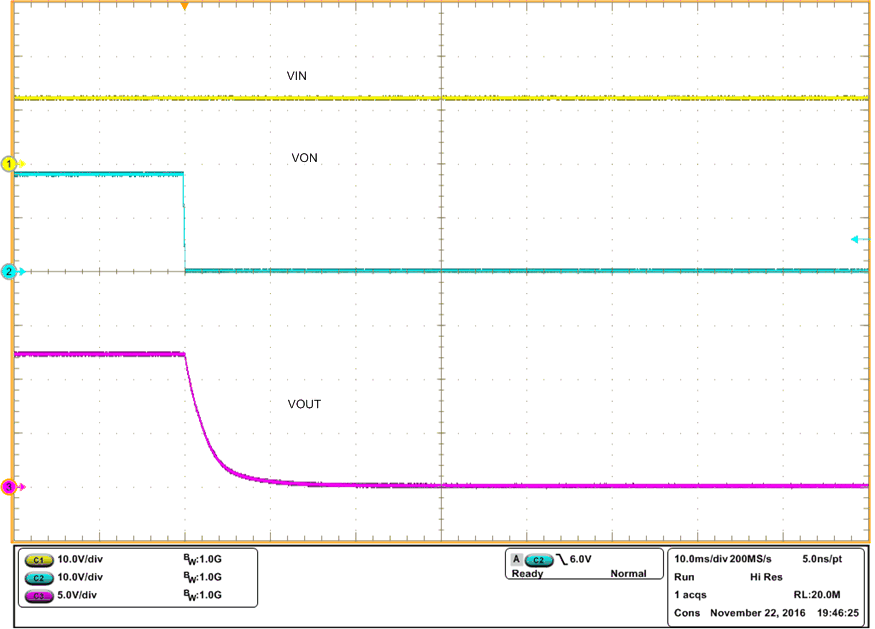VIN = 12 V CIN = 1 µF CL = 10 µF
Figure 24. tF at VIN = 12 V , QOD = VOUTVIN = 12 V CIN = 1 µF CL = 22 µF
Figure 26. tF at VIN = 12 V, RQOD = 500 Ω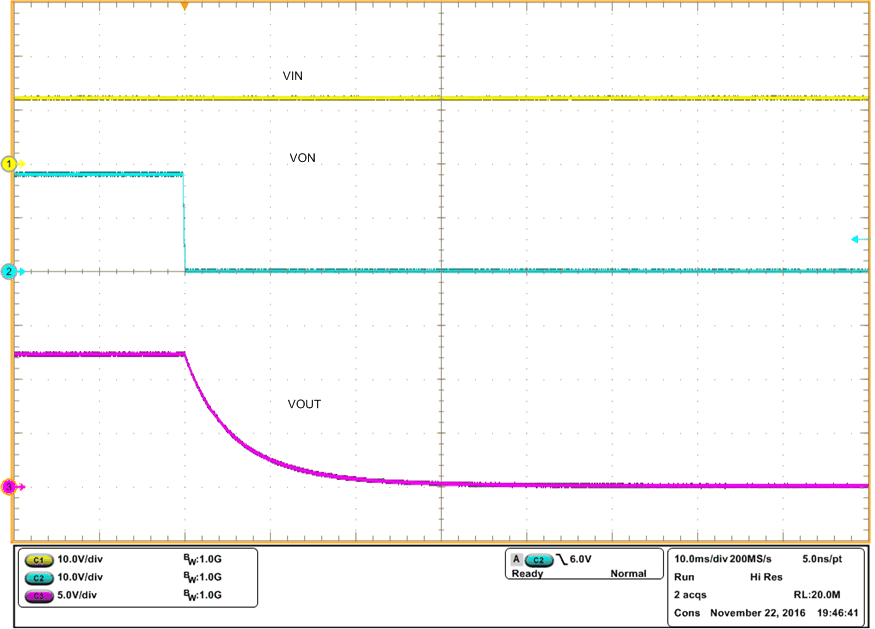VIN = 12 V CIN = 1 µF CL = 10 µF
Figure 23. Fall Time tF at VIN = 12 V, RQOD = 500 ΩVIN = 12 V CIN = 1 µF CL = 22 µF
Figure 25. tF at VIN = 12 V, RQOD = 1000 Ω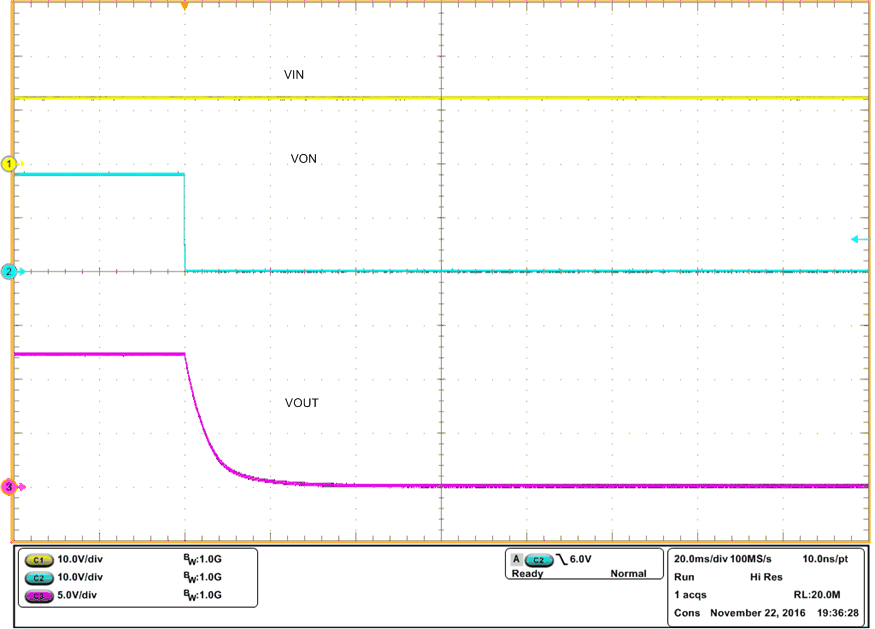VIN = 12 V CIN = 1 µF CL = 22 µF
Figure 27. tF at VIN = 12 V, QOD = VOUT